The CUSUM Procedure

Input Data Sets

DATA= Data Set

You can read raw data (measurements) from a DATA= data set specified in the PROC CUSUM statement. Each process specified in the XCHART statement must be a SAS variable in the DATA= data set. The values of this variable are typically measurements of a quality characteristic taken on items in subgroup samples indexed by the values of the subgroup variable. The subgroup-variable specified in the XCHART statement must also be a SAS variable in the DATA= data set. Other variables that can be read from a DATA= data set include

• _PHASE_ (if the READPHASES= option is specified)

• block-variables

• symbol-variable

• BY variables

• ID variables

Each observation in a DATA= data set should contain a raw measurement for each process and a value for the subgroup variable. If theth subgroup contains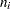items, there should beconsecutive observations for which the value of the subgroup variable is the index of theth subgroup. For example, if each of 30 subgroup samples contains five items, the DATA= data set should contain 150 observations.

By default, the CUSUM procedure reads all of the observations in a DATA= data set. However, if the DATA= data set includes the variable _PHASE_, you can read selected groups of observations (referred to as phases) by specifying the READPHASES= option in the XCHART statement.

For an example of a DATA= data set, see Creating a V-Mask Cusum Chart from Raw Data.

LIMITS= Data Set

You can read cusum scheme parameters from a LIMITS= data set specified in the PROC CUSUM statement.1 As an alternative to specifying the parameters with options, a LIMITS= data set provides the following advantages: it facilitates reusing a permanently saved set of parameters, reading a distinct set of parameters for each process specified in the XCHART statement, and keeping track of multiple sets of parameters for the same process over time.

The LIMITS= data set can be an OUTLIMITS= data set that was created in a previous run of the CUSUM procedure. Such data sets always contain the variables required for a LIMITS= data set; consequently, this is the easiest way to construct a LIMITS= data set.

A LIMITS= data set can also be created directly using a DATA step. The variables required for the data set depend on the type of cusum scheme and how the scheme is specified. The following restrictions apply:

• The variables _VAR_, _SUBGRP_, _DELTA_, and _MU0_ are required.

• For a one-sided cusum scheme, _H_ is required.

• For a two-sided cusum scheme, one of the following three variables is required: _ALPHA_, _H_, or _SIGMAS_.

• If you plan to use the READINDEX= option, the variable _INDEX_ is required; otherwise, it is optional.

• For a one-sided scheme, the variable _SCHEME_ is required; otherwise, it is optional.

• If you want to provide a value for the process standard deviation
, the variable _STDDEV_ is required; otherwise, it is optional.

Variable names in a LIMITS= data set are predefined; the procedure reads only variables with these predefined names. With the exception of BY variables, all names start and end with an underscore. In addition, note the following:

• The variables _VAR_, _SUBGRP_, _TYPE_, and _SCHEME_ must be character variables of length eight. The variable _INDEX_ must be a character variable of length 16.

• The variable _TYPE_ is a bookkeeping variable that uses the values 'ESTIMATE' and 'STANDARD' to record whether the value of _STDDEV_ represents an estimate or standard (known) value.

• BY variables are required if specified with a BY statement.

For an example of reading control limit information from a LIMITS= data set, see Reading Cusum Scheme Parameters.

HISTORY= Data Set

Instead of reading raw data from a DATA= data set, you can read subgroup summary statistics from a HISTORY= data set specified in the PROC CUSUM statement. This enables you to reuse OUTHISTORY= data sets that have been created in previous runs of the CUSUM, MACONTROL, or SHEWHART procedures or to read output data sets created with SAS summarization procedures such as PROC MEANS. A HISTORY= data set must contain the following variables:

• subgroup-variable

• subgroup mean variable for each process

• subgroup standard deviation variable for each process

• subgroup sample size variable for each process

The names of the subgroup mean, subgroup standard deviation, and subgroup sample size variables must be the process concatenated with the special suffix characters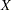,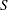, and
respectively.

For example, consider the following statements:

proc cusum history=Steel limits=Steelparm;
xchart (Weight Yieldstrength)*Batch;
run;

The data set Steel must contain the variables Batch, WeightX, WeightS, WeightN, YieldstrengthX, YieldstrengthS, and YieldstrengthN.

Note that if you specify a process name that contains 32 characters, the names of the summary variables must be formed from the first 16 characters and the last 15 characters of the process name, suffixed with the appropriate character.

Other variables that can be read from a HISTORY= data set include

• _PHASE_ (if the READPHASES= option is specified)

• block-variables

• symbol-variable

• BY variables

• ID variables

By default, the CUSUM procedure reads all of the observations in a HISTORY= data set. However, if the HISTORY= data set includes the variable _PHASE_, you can read selected groups of observations (referred to as phases) by specifying the READPHASES= option.

For an example of reading summary information from a HISTORY= data set, see Creating a V-Mask Cusum Chart from Subgroup Summary Data.Previous Page | Next Page | Top of Page